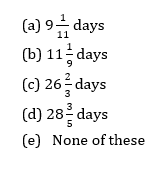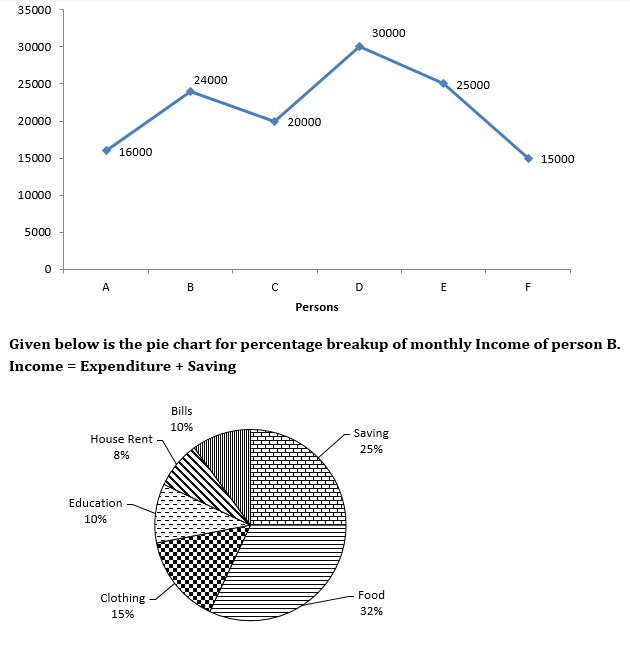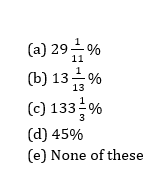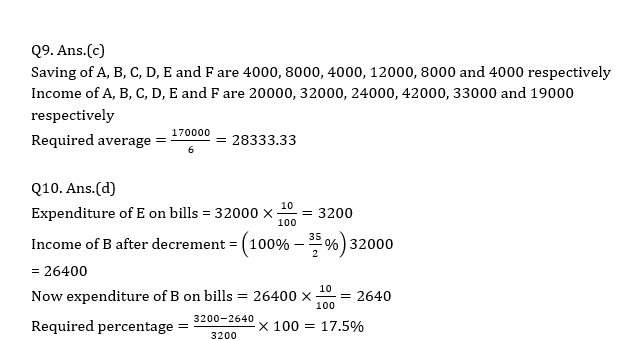# Quant Quiz for IBPS CLERK MAINS 2018 | 10th January 2019

Quant Quiz to improve your Quantitative Aptitude & Data Interpretation. For upcoming exams like Bank, SSC, Railway, UPSC, UPSSSC, CDS, UPTET, KVS, DSSSB and other Government exams.

Q1. Ashok borrowed some money at simple interest at the rate of 6 per cent per annum for the first two years, at the rate of 9 per cent per annum for the next three years and at the rate of 14% per cent per annum for the period beyond five years. If he pays a total interest of Rs. 11400 at the end of 9 years how much money did he borrow ?

(a) Rs. 16,000

(b) Rs. 14,000

(c) Rs. 18,000

(d) Rs. 12,000

(e) None of these

Q2. In an alloy, zinc and copper are in the ratio 1 : 2. In the second alloy the same elements are in the ratio 2 : 3. In what ratio should these two alloys be mixed to form a new alloy in which the two elements are in ratio 5 : 8?

(a) 7 : 11

(b) 3:10

(c) 5 : 11

(d) 9:11

(e) None of these

Q3. To do a certain work B would take 4 times as long as A and C together and C would take 3 times as long as A and B together. The three men together complete the work in 5 days. How long would take B and C to complete the work ?Q4. A man can row at a speed of 4.5 km/hr in still water to a certain upstream point and back to the starting point in a river which flows at 1.5 km/hr. Find his average speed for total journey?

(a) 4 km/hr

(b) 6 km/hr

(c) 4.5 km/hr

(d) 5 km/hr

(e) None of these

Q5. 40 men, working 8 hours a day can do a piece of work in 15 days. Find the number of days in which second group of 60 men working 4 hrs a day can do twice the work. Assume that 3 men of the first group do as much work in 2 hour as 4 men of the second group do in 3 hrs?

(a) 60 days

(b) 40 days

(c) 80 days

(d) 70 days

(e) None of these

Directions (Q6-Q10): Study the following graphs carefully and answer the questions given below:

Graph given below shows the monthly expenditure by 6 personsNote: Consider person B don’t have any other expenditure.

Q6. If ratio of expenditure of person D on food and clothing is 8 : 7 and expenditure of D on clothing is 16 2/3% more than the expenditure of B on clothing, then what is the sum of expenditure  of person D on food and clothing together ?

(a) 10500

(b) 8700

(c) 12000

(d) 14000

(e) None of these

Q7. If D and F spend 20% and 100/3% of their monthly expenditure on house rent then expenditure of B on Electricity is what percent of expenditure of D and F together on house rent (approximately) ?Q8. If income of C is 1100/3% more than expenditure of B on clothing, then saving of C is approximately what percent more or less than saving of B ?

(a) 30%

(b) 40%

(c) 70%

(d) 60%

(e) 35%

Q9. If saving of A, B, C, D, E and F are in the ratio 1 : 2 : 1 : 3 : 2 : 1 then what is the average of their income ?

(a) 30520

(b) 70252.33

(c) 28333.33

(d) 27089.33

(e) None of these

Q10. Expenditure of person E on bill is equal to the expenditure of B on bills. If Income of B is decreased by 17.5% then expenditure on bill for person E is changed by what percent (approximately)?

(a) 20%

(b) 165

(c) 12.5%

(d) 17.5%

(e) 8.5%

# Solutions## WhatsApp Group Join here

Mail us at : ambitiousbaba1@gmail.com